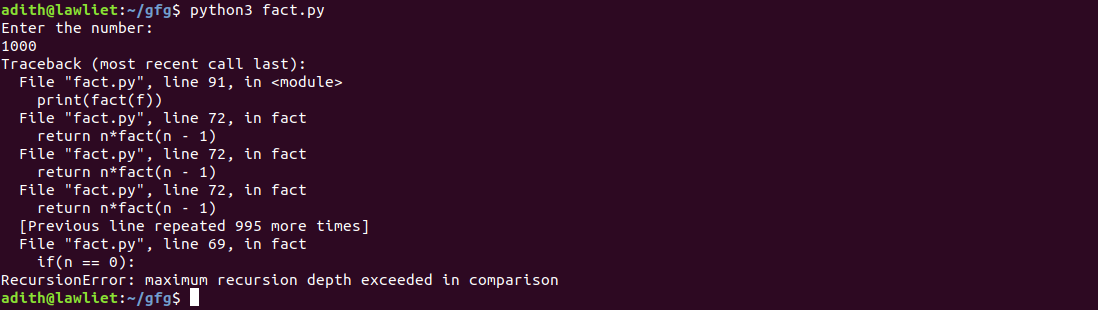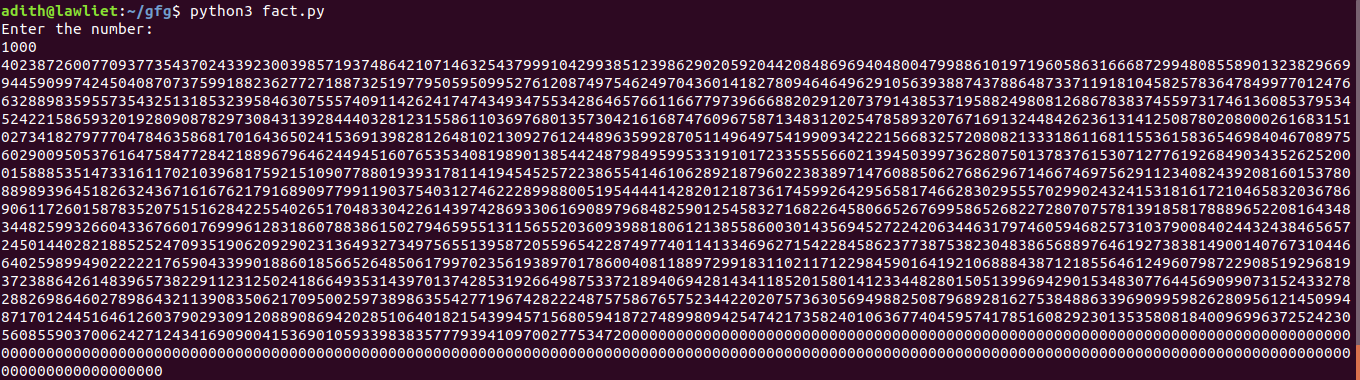# Python | Handling recursion limit

When you execute a recursive function in Python on a large input ( > 10^4), you might encounter a “maximum recursion depth exceeded error”. This is a common error when executing algorithms such as DFS, factorial, etc. on large inputs. This is also common in competitive programming on multiple platforms when you are trying to run a recursive algorithm on various test cases.

In this article, we shall look at why this error occurs and how to handle it in Python. To understand this, we need to first look at tail recursion.

Tail recursion

In a typical recursive function, we usually make the recursive calls first, and then take the return value of the recursive call to calculate the result. Therefore, we only get the final result after all the recursive calls have returned some value. But in a tail recursive function, the various calculations and statements are performed first and the recursive call to the function is made after that. By doing this, we pass the results of the current step to the next recursive call to the function. Hence, the last statement in a Tail recursive function is the recursive call to the function.
This means that when we perform the next recursive call to the function, the current stack frame (occupied by the current function call) is not needed anymore. This allows us to optimize the code. We Simply reuse the current stack frame for the next recursive step and repeat this process for all the other function calls.

Using regular recursion, each recursive call pushes another entry onto the call stack. When the functions return, they are popped from the stack. In the case of tail recursion, we can optimize it so that only one stack entry is used for all the recursive calls of the function. This means that even on large inputs, there can be no stack overflow. This is called Tail recursion optimization.

Languages such as lisp and c/c++ have this sort of optimization. But, the Python interpreter doesn’t perform tail recursion optimization. Due to this, the recursion limit of python is usually set to a small value (approx, 10^4). This means that when you provide a large input to the recursive function, you will get an error. This is done to avoid a stack overflow. The Python interpreter limits the recursion limit so that infinite recursions are avoided.

Handling recursion limit –

The “`sys`” module in Python provides a function called` setrecursionlimit()` to modify the recursion limit in Python. It takes one parameter, the value of the new recursion limit. By default, this value is usually 10^4. If you are dealing with large inputs, you can set it to, 10^6 so that large inputs can be handled without any errors.

Example:

Consider a program to compute the factorial of a number using recursion. When given a large input, the program crashes and gives a “maximum recursion depth exceeded error”.

 `# A simple recursive function ` `# to compute the factorial of a number ` `def` `fact(n): ` ` `  `    ``if``(n ``=``=` `0``): ` `        ``return` `1` ` `  `    ``return` `n ``*` `fact(n ``-` `1``) ` ` `  ` `  `if` `__name__ ``=``=` `'__main__'``: ` ` `  `    ``# taking input ` `    ``f ``=` `int``(``input``(``'Enter the number: \n'``)) ` ` `  `    ``print``(fact(f)) `

Output :Using the `setrecursionlimit()` method, we can increase the recursion limit and the program can be executed without errors even on large inputs.

 `# importing the sys module ` `import` `sys ` ` `  `# the setrecursionlimit function is ` `# used to modify the default recursion ` `# limit set by python. Using this,  ` `# we can increase the recursion limit ` `# to satisfy our needs ` ` `  `sys.setrecursionlimit(``10``*``*``6``) ` ` `  `# a simple recursive function ` `# to compute the factorial of a number ` `# it takes one parameter, the  ` `# number whose factorial we  ` `# want to compute and returns ` `# its factorial ` `def` `fact(n): ` ` `  `    ``if``(n ``=``=` `0``): ` `        ``return` `1` ` `  `    ``return` `n ``*` `fact(n ``-` `1``) ` ` `  `if` `__name__ ``=``=` `'__main__'``: ` ` `  `    ``# taking input ` `    ``f ``=` `int``(``input``(``'Enter the number: \n'``)) ` ` `  `    ``print``(fact(f)) `

Output :My Personal Notes arrow_drop_upCheck out this Author's contributed articles.

If you like GeeksforGeeks and would like to contribute, you can also write an article using contribute.geeksforgeeks.org or mail your article to contribute@geeksforgeeks.org. See your article appearing on the GeeksforGeeks main page and help other Geeks.

Please Improve this article if you find anything incorrect by clicking on the "Improve Article" button below.

Article Tags :

2

Please write to us at contribute@geeksforgeeks.org to report any issue with the above content.